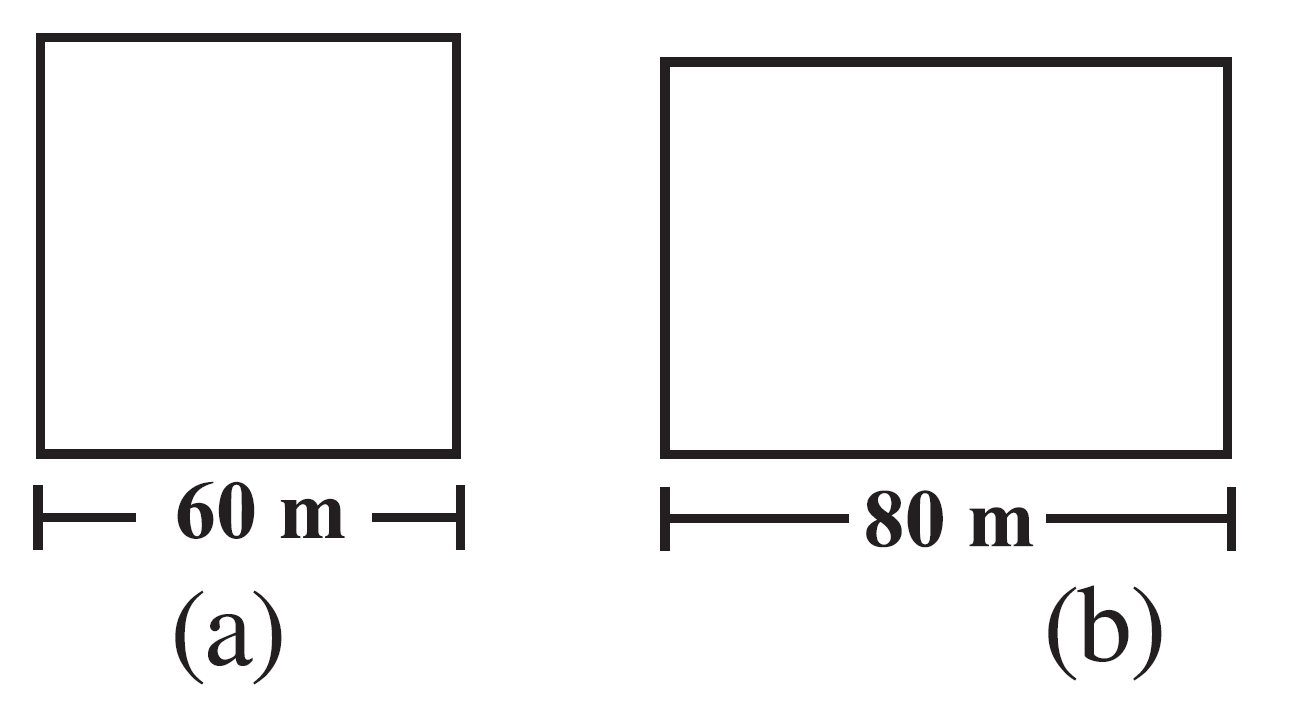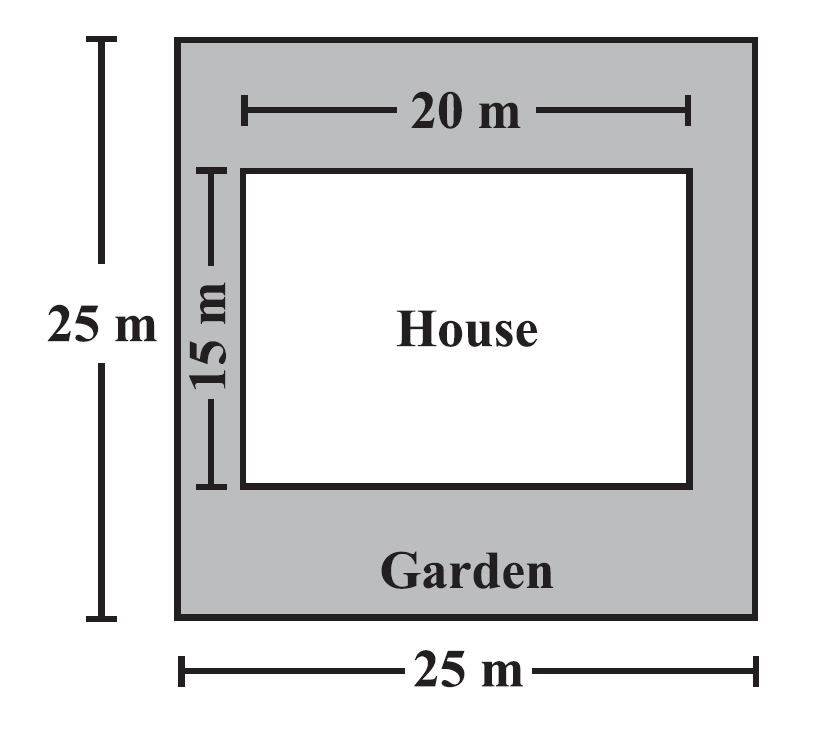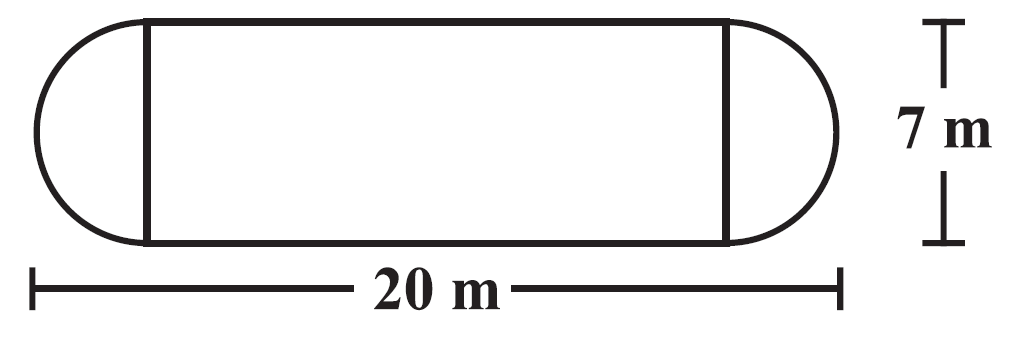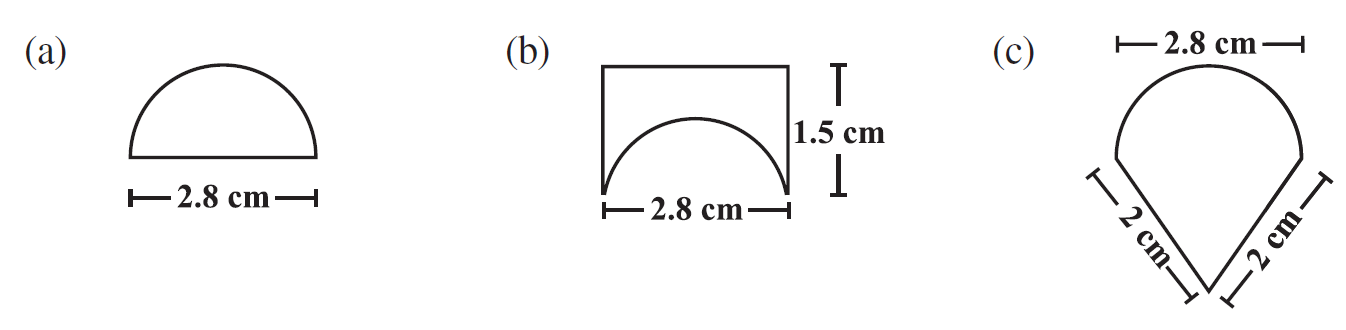# vs.eyeandcontacts.com

## Chapter 11 Mensuration Exercise 11.1

Question 1: A square and a rectangular field with measurements as given in the figure have the same perimeter. Which field has a larger area?Given
Side of square = 60 m
Rectangle length = 80 m
Square and rectangle have same perimeter.
To find
Which field has a larger area?
Formula
Perimeter of square = 4 × s
Perimeter of rectangle = 2(l + b)
Area of square = s²
Area of rectangle = l × b
Working
= 4 × s = 2(l + b)
= 4 × 60 = 2(80 + b)
= 240 = 160 + 2b
= 2b = 240 - 160
= 2b = 80
= b = 40 m
Area of square = ?
= s × s
= 60 × 60
= 3600 m²
Area of rectangle = ?
= l × b
= 80 × 40
= 3200 m²
Comparing
3600 m² > 3200 m²
Therefore, square has a larger area.

Question 2: Mrs. Kaushik has a square plot with the measurement as shown in the figure. She wants to construct a house in the middle of the plot. A garden is developed around the house. Find the total cost of developing a garden around the house at the rate of Rs 55 per m².Given
Length of side of square plot = 25 m
Length of house = 20 m
Breadth of house = 15 m
Cost of developing garden around the house per m² = ₹55
To find
The total cost of developing a garden around the house.
Formula
Area of square = s × s
Area of rectangle = l × b
Working
Area of square plot = ?
= 25 × 25
= 625 m²
Area of house = ?
= 20 × 15
= 300 m²
Area of garden = Area of square plot - Area of house
= 625 - 300
= 325 m²
Cost of developing garden around the house = ?
= 325 × 55
= ₹17875
Therefore, the cost of developing garden around the house is ₹17875.

Question 3: The shape of a garden is rectangular in the middle and semi circular at the ends as shown in the diagram. Find the area and the perimeter of this garden [Length of rectangle is 20 - (3.5 + 3.5) metres].Given
Shape of garden is in the form of rectangle in middle and semi circle at the ends.
Rectangle
length = 13 m
Radius of circle = 3.5 m
To find
The area and the perimeter of this garden
Formula
Perimeter of rectangle = 2(l + b)
Perimeter of circle = 2πr
Area of rectangle = l × b
Area of circle = πr²
Working
Area of park = ?
= Area of semi circle + Area of rectangle + Area of semi circle
= πr²/2 + l × b + πr²/2
= πr² + l × b
= [22/7 × (3.5)²] + [13 × 7]
= [22/7 × 35/10 × 35/10] + 91
= 38.5 + 91
= 129.5 m²
Perimeter of park = ?
= Perimeter of semi circle + Length of rectangle + Length of rectangle + Perimeter of rectangle
= 2πr/2 + l + l + 2πr/2
= 2πr + 2(l)
= [2 × 22/7 × 3.5] + [2(13)]
= [2 × 22/7 × 35/10] + 
= 22 + 26
= 48 m
Therefore, the perimeter of park is 48 m while the area of rectangle is 129.5 m².

Question 4: A flooring tile has the shape of a parallelogram whose base is 24 cm and the corresponding height is 10 cm. How many such tiles are required to cover a floor of area 1080 m²? (If required you can split the tiles in whatever way you want to fill up the corners).

Given
Flooring tile in form of parallelogram
base = 24 cm
height = 10 cm
area of floor = 1080 m²
To find
The number of tiles required to cover the floor
Formula
Area of parallelogram = b × h
Working
Area of one tile = ?
= 24 × 10
= 240 cm²
Number of tiles required = ?
= 1080 m²/240 cm²
= 10800000/240
= 1080000/24
= 45000 tiles
Therefore, 45000 tiles would be required to cover the floor whose area is 1080 m².

Question 5: An ant is moving around a few food pieces of different shapes scattered on the floor. For which food-piece would the ant have to take a longer round? Remember, circumference of a circle can be obtained by using the expression c = 2πr, where r is the radius of the circle.a)
Distance covered by the ant to collect food pieces = ?
= 1/2 × 2πr + 2r
= πr + 2r
= 22/7 × 1.4 + 2 × 1.4
= 22/7 × 14/10 + 2.8
= 22/7 × 14/10 + 28/10
= 44/10 + 28/10
= 72/10
= 7.2 cm
b)
Distance covered by the ant to collect food pieces = ?
= 1.5 + 1.5 + 2.8 + 1/2 × 2πr
= 5.8 + πr
= 5.8 + 22/7 × 1.4
= 5.8 + 4.4
= 10.2 cm
c)
Distance covered by the ant to collect food pieces = ?
= 1/2 × 2πr + 2 + 2
= πr + 4
= 22/7 × 1.4 + 4
= 4.4 + 4
= 8.4 cm
Comparing
10.2 cm > 8.4 cm > 7.2 cm
Therefore, for b) food-piece, the ant have to take a longer round.